Adapted for the Internet from:Why God Doesn't Exist

1.0   Constructing continuity with discrete building blocks

One of the strategic terms that is responsible for much misunderstanding in Mathematics is the word
continuous. In 3000 years, the mathematicians have never been able to discover the contradictions they
built into this enigmatic word.

In Mathematics, 'continuous' is a property having to do with how close two discrete points are to each other
on a line:

" continuous: A general mathematical property obeyed by mathematical objects in
which all elements are within a neighborhood of nearby points" 

" we endeavour to trace back all conceptions of continuity to the conception
'between,' i.e. to the relation A is a point of the straight line BC and lies between
B and C."  (p. 12) 

" the function is continuous at any point where it does not 'skip' from one value to
a distant value, but instead 'changes smoothly.' " 

" continuum: A compact connected set. If a set contains at least two points, it contains
an infinite number of points, since between any two points there is a third point." 

Mathematical continuity has to do with the degree of smoothness, with the notion of 'connectedness.' Thus:

The finer the grains and the closer they are to each other, the smoother the pile
of sand and the more 'continuous' is the slope of the dune.
If temperature rises gradually, the function is said to be continuous.
If you constantly deposit and withdraw money from your bank account, the function
is said to be discontinuous.

And so on.

However, if the object is comprised of units, how can it also be uninterrupted? Again the mathematicians have
always wanted it both ways to peddle their theories. Mathematics thrives on dualities.

I have no problem with this poppycock as long as it stays within the confines of Math. The trouble is that the
Mathematicians tend to extrapolate their ridiculous notion to the physical world and purport to call this
impossible mixture 'science' or Geometry or something like that:

" continuous: Attached together in repeated units." 

" The continuity of space means that space can be divided up into subregions."  

" The basic idea behind geometric continuity was that the five conic sections were
really five different versions of the same shape; it was possible to construct ellipses
towards the limit of either a circle or a parabola, and likewise to construct hyperbola
that approached the shapes of a parabola or a straight line. Thus, there was continuity
between the conic sections."  

" a row of points suggested by a tightly stretched string, the edge of a desk, or a
flagpole, extended infinitely in both directions, is called a line."  

" Notice what a geometrical interpretation of the final conclusion could be: a line
of infinite length (the real number line) has the same number of points as any
finite line." 

" We assume that space is continuous, that there are no breaks in it. The positions
occupied by a body travelling from any one place to any other form a perfectly
connected series. If they did not, either the body would cease to exist, or would be
outside our space at the gaps. The Postulate of Continuity states that two adjacent
portions of space have the same boundary, which is a surface, a line, or a point,
in the cases respectively of two adjacent portions of a solid, a surface, or a line.
Between any two points on a line there may be an infinite number of intermediate
points."  

" A continuum is a body that can be continually sub-divided into infinitesimal small
elements" 

" In physics, a rigid body is an idealization of a solid body of finite size in which
deformation is neglected. In other words, the distance between any two given
points of a rigid body remains constant regardless of external forces exerted
on it." 

[No. This is the mathematical definition of rigid!]

Here we see how different Math is from Physics and why Math is not the language of Physics. In Physics and
in Science, continuous means exactly the opposite of what it means in Mathematics (Fig. 1):

continuous (adj.): A property of a hypothetical, indivisible object. A word used
to describe a hypothetical object made of a single piece or unit.

In Physics, rigid means 'single piece':

" If all the parts making up a medium were rigidly bound, then they would all vibrate
as one, with no delay in the transmission of the vibration and therefore no wave
motion (or rather infinitely fast wave motion)." 

Only then is the object conceptually rigid. There are no rigid objects in the real world. Rigid is a word used in
idealized situations. Rigid is also not a matter of degrees as in 'this object is more rigid than that one. This
statement belongs to ordinary speech. Either an object is rigid or it isn't. Only then can we use the adjective
rigid  consistently (i.e., scientifically).

In Physics, if the proponent alleges that a figure is made of parts or segments or discrete points, he cannot
also say that it is continuous. For the purposes of Science, the word continuous is exclusively an adjective.
This means that we can only use it to qualify physical objects, for example, the line of Geometry. Again, this
is the only manner in which we can use the adjective continuous consistently            (i.e., scientifically).
Concepts such as the amount of money in your bank account may not be said to be continuous, for what
meaning could this contradiction have? And if each grain of sand is discrete, the dune you build with it is
definitely not continuous for the same reason. A hill is continuous only if it is not made of parts, meaning
that we find no pockets of empty space within it. Of course, pursuant to this definition, not a single physical
object in the macro world is continuous. Like the adjective rigid, continuous may only be used in idealized
situations.

The nature of the problem is really that the mathematicians are attempting to use the word continuous as an
adverb. The correct word is not the adjective continuous, but the adverb incessant. The mathematician is
saying that he can incessantly generate numbers between any two numbers, or that you incessantly withdraw
money from your bank account, or that temperature incessantly increases. In Science, we use adverbs to
qualify motion. The idiots of Mathematics have borrowed language from ordinary speech and unjustifiably
ushered the words into scientific contexts. Thus, they say straight line, when they really mean rectilinear
itinerary or infinite numbers (or infinity) when they really mean incessant counting. For the purposes of
Science, the adjective incessant of ordinary speech is strictly an adverb. Jumping can perhaps be said to be
incessant. A standalone cube cannot be incessant.
 Is a line continuous or segmented?And a wing is continuous if it is made of many feathers. See? I can hang from the stupid swan's wing and it won't break.
 Fig. 1   Continuous mathematical misconceptions
 In Mathematics, the word 'continuous' means exactly the opposite of what it means in Science. In Math, continuous means that the object or concept is comprised of so many discrete objects or concepts that it 'approaches' physical or conceptual smoothness. This definition is unscientific because it cannot be used consistently.For the purposes of Science, 'continuous' means that the thing in question is made of a single piece. The adjective 'continuous' may only be used to qualify physical objects. The real problem is that the mathematicians are using the adjective continuous as an adverb. They are talking about motion and not about architecture. The correct word to characterize what they are referring to is incessant. The mathematicians are saying that they can incessantly find fractions between anytwo.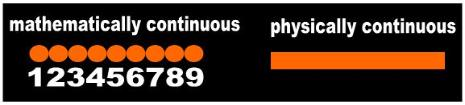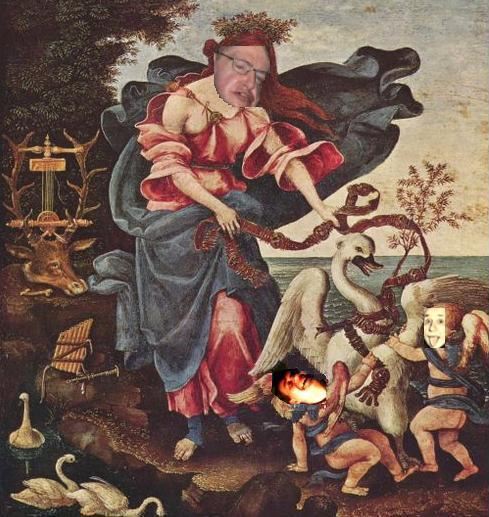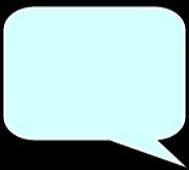A belt is continuous if it is made of many small pieces. See? I can strangle this swan with it and the belt won't break.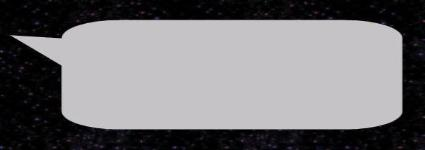Ha, ha, ha! You two continuously make me laugh! That swan you're messing with turns into a vicious dragon every time someone bothers it. He just finished trashing that deer behind me a minute ago!

5.0   Can motion be continuous?

Another serious mistake the mathematicians make is the claim that a function can be continuous. They
routinely use the word continuous in the context of motion:

" An intuitive though imprecise (and inexact) idea of continuity is given by the
common statement that a continuous function is a function whose graph can
be drawn without lifting the chalk from the blackboard."  

" Uninterrupted in time, sequence, substance, or extent."  

" Current electricity is a continuous flow of electrons through a circuit." 

[The author is not saying that the ‘object’ electron is internally indivisible
(i.e. continuous). He is saying that the flow of electrons is 'incessant.']

" Of or relating to a line or curve that extends without a break or irregularity." 

A function only has to do with motion, and so the natural question is whether motion can be continuous.

The first problem again is language. In Science, the word continuous may only be used to qualify physical
objects (§ 1.0). This summarily eliminates dynamic concepts such as motion or function. We're done!

But let's overlook this limitation for the moment and assume that we can extend the use of this strategic
word to concepts as well. Can motion now be said to be continuous?

Motion requires two or more locations of an object. In familiar terms, we are watching two or more frames of
a movie. The unique locations an object can occupy appear in different frames. Indeed, an object cannot be
at two different locations. When it is here, it isn't there and vice versa. Therefore, by definition motion is
necessarily a segmented concept, meaning that it is not 'continuous'. Motion is a movie and irrespective of
how detailed it is, a movie is made of discrete frames. In each frame we see the object only once. Hence,
memory misleads us to believe that motion is continuous. This is an observer-related issue. The stone flying
through the air can only be at one location in each frame of the film. Motion is what the stone does and not
what the observer infers.  There is no such notion as continuous motion even in the case where we were
allowed to use the adjective continuous in tandem with a word depicting motion. The best we can say is that
motion is incessant, perpetual, or constant. These are the correct words to use in the context of motion. The
phrase 'continuous motion' is unscientific. It belongs exclusively in ordinary speech together with the related,
popular phrase concocted by the mathematicians: 'continuous function'. It is the mathematicians’ casual use
of scientific adjectives as adverbs of ordinary speech that leads to the ridiculous statements we routinely
read in the 'scientific' literature.'

6.0   'Continuous' set of points or two points?

Nevertheless, if the 'continuous' set of points definition holds, it instantly debunks the argument that two lonely
points in space constitute a straight line. If to be straight, a line must be comprised of a continuous set of
points, the two isolated points do not yet meet the ‘continuous’ requirement. In fact, it is easy to show that
they never will. Were the points to touch nose to nose, they would still be unable to blend and become
continuous without violating our hypothesis.  To remain discrete, space must delineate the entire contour of
a point. If the points fuse to form a continuous entity, we would be unable to talk about points or conceive of
'between'. Again, we are interested in Physics and not in the idiotic religion Mathematics has become.

4.0   The vector

One major problem the mathematicians run up against with continuity is their notion of the vector. A vector is
supposed have only two properties: magnitude and direction.

" vector: a concept characterized by a magnitude and a direction" 

Magnitude is more or less a synonym of size and amount.  In order to talk about it the mathematician
invokes the number line. Magnitudes are quantities --  numbers --  and by their very nature discrete. Typical
examples of magnitudes are real and complex numbers, fractions, and line segments.  On the other hand,
direction is inherently made of a single piece. We cannot chop direction in half for fear of offending God!
Therefore, the infamous vector of Mathematics has merged two irreconcilable concepts: the segmented
magnitudes of Mathematics and the qualitative, single-piece direction of Physics (Fig. 3). For the purposes of
Science, direction is conceptually 'continuous' (within the context just discussed -- only physical objects may
be truly said to be continuous in Science). This just goes to show that Mathematics and Physics don't blend!
Numbers have absolutely nothing to do with the physical world!
 Fig. 3   The 'continuous' vector of Mathematics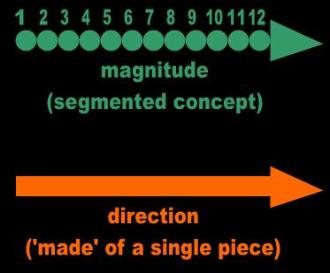The vector of Mathematics is an attempt to merge two irreconcilable concepts: magnitude and direction.Magnitude essentially means size or amount.It is inherently a segmented concept. In this context, it refers to numbers on a number line,each of which is a unique value.Direction is conceptually made of a single piece. It makes no sense to divide directionin half.The mathematicians have incongruouslymerged the discrete with the continuous.
 Fig. 2   The supernatural mathematical line
At the start of His presentation, God points to a series of dots and
calls it a line. He then takes His magic wand, and converts it into a
rigid stick made of a single piece. Throughout his presentation,
God talks about numbers and locations. Sounds like a plan?

Just in case, locations had also better be discrete. How else are we are to distinguish them from one another?
Without an object occupying it, a location is nothing. For the purposes of Science, all objects are by definition
discrete. We measure distance from the edge or surface of an object. Hence, a series of locations are
conceptually segmented. Otherwise, we would talk about a single location.

Hence, two dots, locations, or numbers can never constitute a continuous line without violating their
individuality irrespective of how they are distributed, aligned, or shaped. We simply cannot construct a
continuous wall using discrete bricks.  If continuity is a precondition for straightness, to be straight, a line
must be an indivisible, continuous entity from the start.

Corollary:

Aristotle incongruously referred to that which cannot be divided as ‘indivisible’ and
to that which could be divided as ‘continuous.’   In Math, indivisible and continuous
are antonyms. In Physics, these terms are synonyms. In Physics, it is irrational to
say that you have constructed a continuous wall using discrete bricks.
 Fig. 4
 The mathematicians routinely tell you that two dots in space constitute a straight line. Unfortunately, this definition or characterizationis misconceived. If the line of Mathematics is continuous and straight, this specification automatically rules out the two-dot proposal.Two dots cannot be said to be continuous orstraight under any circumstances.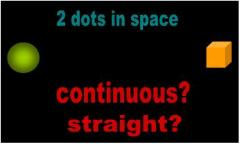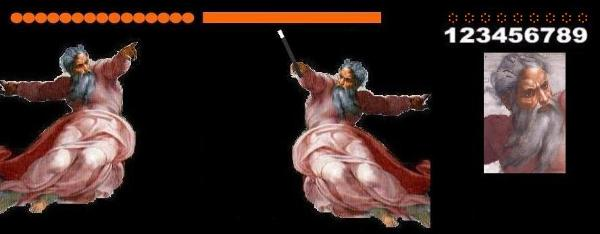2.0   In Math, discrete means continuous and continuous means discrete

Ironically, the mathematicians have developed such idiotic language that they have confused themselves
beyond hope. The notions and definitions of Mathematics are not only unscientific, but also exactly opposite
of what they mean in Physics. One mathematician attempts to establish the difference between continuous
and discrete:

" let us distinguish what is continuous from what is discrete. A natural number
is a collection of discrete units. 5 pencils, 8 electrons, 100 people.  Each one  is
indivisible.  You cannot take half of any one.  If you do, it will not be that unit
-- that same kind of thing -- any more.  Half a person is not also a person! But
consider the distance between A and B.  That distance is not made up of
discrete units.  It is not a number of anything.  We say, instead, that it is
continuous.  That means that as we go from A to B, there is no break.
(Continuous does not mean stretches on and on -- "continues"; it means not
composed of discrete units.  Thus if you study continuously for an hour, you
do not take a break.)" 

If this is what the mathematicians understand by the adjectives continuous and discrete, it once again
underscores that Mathematics is NOT the language of Physics. The mathematicians have incongruously
concluded that whatever is made of parts is continuous and whatever cannot be divided is discrete. For
the purposes of Science, continuous is that which cannot be divided in particular because it is not made
of discrete parts. This is the scientific version because it can be used  consistently. The opposite of
continuous is not the word discrete, as Spector insinuates, but the word segmented. Discrete is a synonym
of object or of shape or of island. Discrete simply means that the object is contoured all around. It has
nothing to do with divisibility.

But more revealing of the ludicrous notions developed by the mathematicians are the examples Spector
gives. He talks about 'units', implying that he is alluding to shapes. In fact, all the words he invokes to
exemplify discreteness --  pencils, electrons, and people -- are physical objects. Suddenly, out of the blue,
Spector gives two examples of continuity that have nothing to do with objects. In one scenario he invokes
the word distance -- which as we all now know means anything but distance. Spector talks about continuous
motion from A to B. In the other, he mentions continuous studying.

So I will make it as clear as I can: You have to be a mathematician to use an adjective in the context of motion.
In Science, we use adjectives to modify physical objects and adverbs to qualify motion. Only then can we use
them consistently (i.e., scientifically). It is unscientific to say that you study continuously. In Science, we say
that you study incessantly, or perpetually, or constantly.

So, clearly, Spector is not talking about anything related to Physics. First and foremost, Physics studies
objects, in particular those objects which exist. Before we can study verbs, we must absolutely have a noun.
So he ends up with the strangest contradictions. Studying is continuous despite that motion is conceptually
segmented (§ 5.0 below), and the line from A to B is continuous despite that the line of Mathematics is
comprised of discrete points!

3.0   The 'continuous' mathematical number line

So where did the mathematicians get their notion of continuity?

In order to answer this question, we need to review the infamous number line of Mathematics, a concept that
not a single mathematician on Earth has ever conceptualized properly, researched, or understood. On the
one hand the mathematicians tell us that the number line is continuous:

" Real numbers measure continuous quantities." 

" the number line is continuous" 

On the other, Spector just finished defining the adjective continuous as 'not composed of discrete units'.
Clearly, every number on the number line is conceptually at least a discrete unit:

" even with an infinite number of numbers between, for example, the numbers 1
and 2, we must believe that each of these numbers (all infinity of them) is
DISCRETE, yes?  Each is separate, and can be no other, no matter how tightly
packed against its infinite brethren... If each point in a line could be named with
its number, each would have its accompanying gap on either side. There must
be a gap or they would 'blend' into some monstrous single number." 

So who is right? Is a number line made of countless discrete numbers (meaning that it is conceptually
segmented or is it made of a single piece?

The answer is that the number line of Math is not a physical  object or geometric figure. The number line is an
abstract concept.  If the word continuous may only be used in the context of physical objects, this summarily
excludes the number line. We're done!

But let us assume that we could use the adjective continuous in Mathematics. What are the mathematicians
referring to when they say that the number line is continuous? Where did they get this ridiculous idea? It is
axiomatic that the number line is comprised of numbers, each of which is conceptually unique, a stand-alone
'entity' differentiated from its neighbor. Therefore, it would not matter how many numbers you wish to place
between any two, the entire sequence would neither be continuous nor approach anything resembling
continuity. By its very nature, the number line is a segmented concept. As Spector himself admits, continuous
means 'not composed of discrete units.' This summarily rules out the number line by definition.

What has happened is that the idiots of Mathematics have concocted the ridiculous notion that the more
numbers we insert between any two makes this segment of the number 'line' less chopped up or segmented.
The reasoning goes something like this:

The mathematicians first visualize a series of numbers as a line. They never
justified where they got this incongruous notion. What does a number have
to do with a geometric figure? Is a number the same thing as a point? Does
it make sense to replace a number with a dot?
The mathematicians next extrapolate this train of thought to the next level
and conclude that a number represents a location on this line, another
claim they fail to justify. We are now two orders removed from logic: from
number to dot and from dot to location.
Finally, the mathematicians imagine finer and finer dots making up the line
as we increasingly squeeze them to make room for more numbers
(i.e., locations, i.e., points).

The mathematicians conclude that the smaller the 'point' (meaning the location, meaning the number) the
more dots they can fit between any two. Since a number can be made as tiny as our imagination wishes, it
follows in their tiny minds that a location, meaning a dot, can also be made as tiny as they want. They call this
contraption the infinitesimal point. The mathematicians conclude that the finer the point, the more material
and the less empty space a line has. From an observer's point of view, the line seems to be morphing into a
solid, 'continuous' entity.

So what are the mathematicians really talking about?

The mathematicians have incorporated three irreconcilable notions into one. They are talking about numbers
(i.e., counting), locations, and dots. They are jumping back and forth between concepts (numbers and
locations on the one hand) and objects (dots and lines on the other).

And still it fails! We still end up with a bunch of discrete dots lined up in a row. A line that is alleged to  be made
of discrete dots cannot be continuous. There must be space delineating and contouring each dot if they are to
preserve their individuality. Otherwise we couldn't talk about dots making up a line. The scholars are aware of
this.

So what did our mathematicians do to get around this shortcoming? They waved their magic wands and
converted the sequence of discrete points into a single stick:

" all the points which we obtain finally fuse together into a linear continuum, in which
they become embedded, giving up their individual existences" (p. 16) 

We went from numbers to locations to dots and still couldn't get continuity. So the mathematician now invokes
magic, melts the physical dots, and blends them into a rigid line: a stick made of a single piece. We have no
more dots. The mathematician amended his initial assumptions. He sacrificed logic in his pursuit of the
definition of continuity.

But the mathematician is unhappy with the results. He can't use this contraption in Mathematics. As soon as he
attempts to do something with this 'continuous,' rigid line he must return to discrete dots, locations, and
numbers:

" we are enabled through these axioms to banish continuity, which is so difficult to
fix precisely, from the logical structure of geometry... the end-points of all vectors
OP... form a straight line" (p. 17-18) 

So we did all this work to define what continuity means only to conclude that continuity is incompatible with the
purposes the geometer has in mind, and he goes back to a line made of points, meaning locations, meaning a
bunch of numbers (Fig. 2)! The mathematicians goes back to his segmented number line.  He calls the entire
exercise 'doing Physics' or 'doing Science' or logic or whatever they do in Mathematics.________________________________________________________________________________________